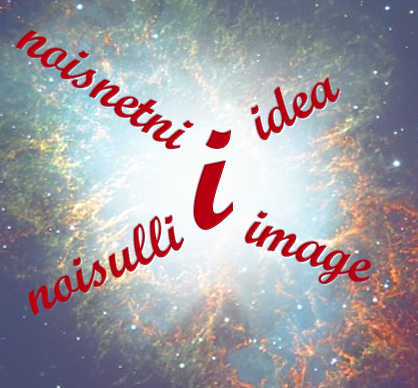# Something from Nothing

Tags: idea

Finally I've decided to blog-out my - still not mature - thoughts on how Something can be created from Nothing.It's all about philosophy and Math, but I'll try to keep it simple, as the most fundamental things in life are simple.

Also keep in mind that what follows is not necessarily 'true', but more a 'thought model' helping to explain and to grasp the phenomenon of creating 'something out of nothing'.

Basic Thoughts
Try to imagine Nothing....

After a few minutes of philosophizing you'll notice, it's not possible to imagine Nothing. All we can come up with, is the 'idea' of Nothing, as Nothing is in fact 'No Thing'.

Now, this idea is exactly what 'was', 'is', and for ever 'will be': an 'idea'. Not a specific idea, but the concept of an idea. That's enough. The concept of an idea  doesn't exist in space or time, it's simply there. It's the metaphor of the concept of Nothing. It's potential.'Idea' is the only word that not only is defined as a noun (passive, object), but 'at the same time' also as a kind of verb (operator, action), as an idea is 'that what can be imagined'. You also 'have' an idea.

Hence, an idea is passive and active at the same time. In self-reference terms the idea is the imagination of an idea.

This idea is in fact what we call 'spirit' in the wider sense of the word. It's an intention, it has the 'potential' (power) of 'creativity', of creating a (positive) illusion. In other words: it's the basis of 'creation'.

Let's define this general concept of  'idea' by the symbol 'i'.

The idea Mathematically expressed
As an idea is 'not real' but imaginary, the most logical mathematical translation of an idea (i) is the mathematical unit imaginary number, denoted as well as: 'i'

'i' is a so called 'complex number' in mathematics with the core property that if you multiply it with itself, the product is "−1".

So i 2 = −1.

As we all now there's no real number that multiplied with itself is a negative number (−1). So (i) will do perfectly as mathematical translation, as we'll see further on.

Next Step: How to create 'Something'
Now we've accepted the general concept of an idea (i), the question is : how to get from an idea (of Nothing) to Something if we have Nothing else but an idea (i).

The problem, or better expressed 'our luck' is that we have nothing else but an idea (i). Fortunately the concept of the idea (i) is as well singular as (not literally) plural and also object as well as operator, as the concept of ideas is still an idea and the imagination of an idea is also an idea.

So all it takes for an idea to create a 'next stage idea' is to apply the idea-concept on itself as an object and operator. It doesn't matter which one is the object or the operator, as object and operator on the idea-level are the same.

Creating Antimatter
O.K. Let's demonstrate what happens if we imply (the concept of) an idea on itself while applying some basic simple 'complex mathematics' as defined above:

i i = i(i) = i 2 =  −1

As we can see, the result is a 'real number'. Not only is it real, but it's also negative.
We can and will interpret a positive real number as what we perceive in our world as 'space'. We can touch it and see it, it's real...
Therefore a negative real number can be interpreted as 'antimatter'.

So applying an idea on itself generates 'antimatter'

Creating Time
Now let's go on by applying an idea on antimatter
Here it is:

i i i(i(i)) = i(i 2) = i(−1) = −i

This result ' −i' can be interpreted as 'time', as is also the case in the definition with regard to space-time by Minkowski.

As 'time'  = i , we can also express time as a kind of idea (thought) in the past (as the sign of time is negative). In other words (free interpreted): time is the perception of thoughts in the past.

Creating Space
Now, in the last step we finally we can apply the concept of idea on 'time', resulting in:

i i i(i i i) = i(-i) = −i 2 = 1

This result can - as defined - be interpreted as 'space' or 'matter'.

So 'space' or 'matter' is more or less the result of thinking or thoughts about time.
In other words, 'space' or 'matter' is our perception (= projected idea) of time in the 'present'.

Applying the idea on space again [ i(1) = i ] would result in the concept of 'idea' again, which starts the cycle of creation from beginning again.

Conclusion
As I've demonstrated by applying a very simple model, we can construct a thought model that subsequently creates antimatter, time and space from the basic imaginary general concept of an 'idea'.So all we need for creation is to apply and multiply our ideas.

Realize and appreciate that you as a human being are also a 'divine' product of idea-creation. By applying  your ideas in the real world, you contribute to this 'world'. Hopefully a 'better' world in line with the idea ..... Just Do It!

Hope you liked this 'philosophy blog'. Please comment your thoughts and ideas on this!

This post first appeared on Selfbetterment, please read the originial post: here

# Share the post

Something from Nothing

×